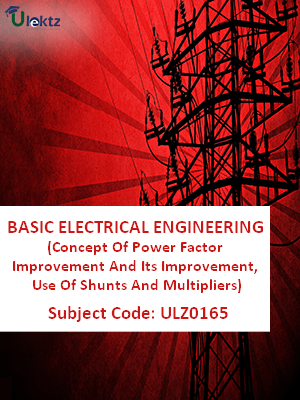•My WalletMy Order
•My Profile
•My Connections
•My Books
•My Videos
•My Tests
•My Calender
•My Messages
•My Shopping Cart
•My Orders
•Account Settings
•Help

# Book Details# Basic Electrical Engineering (Concept Of Power Factor Improvement And Its Improvement, Use Of Shunts And Multipliers)

 Course Code : ULZ0165 Author : uLektz University : General for All University Regulation : 2017 Categories : Mechanical Format :ePUB3 (DRM Protected) Type : eBook

FREE

Description :Basic Electrical Engineering (Concept Of Power Factor Improvement And Its Improvement, Use Of Shunts And Multipliers) of ULZ0165 covers the latest syllabus prescribed by General for All University for regulation 2017. Author: uLektz, Published by uLektz Learning Solutions Private Limited.

Note : No printed book. Only ebook. Access eBook using uLektz apps for Android, iOS and Windows Desktop PC.

##### Topics
###### UNIT -I ELECTRICAL CIRCUIT ANALYSIS

1.1 Introduction, Circuit Concepts: Concepts of network, Active and passive elements, Voltage and current sources

1.2 Concept of linearity and linear network, Unilateral and bilateral elements, Source transformation

1.3 Kirchhoff’s laws

1.4 Loop and nodal methods

1.5 Star-delta transformation

1.6 AC fundamentals: Sinusoidal, square and triangular waveforms, Average and effective values, Form and peak factors, Concept of phasors, Phasor representation of sinusoidally varying voltage and current

###### UNIT - II STEADY- STATE ANALYSIS OF SINGLE PHASE AC CIRCUITS AND NETWORK THEOREMS

2.1 Analysis of series and parallel RLC Circuits, Concept of Resonance in series & parallel circuits

2.2 Bandwidth and quality factor, Apparent, active & reactive powers

2.3 Power factor, Concept of power factor improvement and its improvement (Simple numerical problems)

2.4 Network theorems (AC & DC with independent sources): Superposition theorem, Thevenin’s theorem, Norton’s theorem, Maximum Power Transfer theorem (Simple numerical problems)

###### UNIT - III THREE PHASE AC CIRCUITS AND MEASURING INSTRUMENTS

3.1 Three phase system-its necessity and advantages, Star and delta connection, Line and phase voltage/current relations, Balanced supply and balanced load

3.2 Three-phase power and its measurement (simple numerical problems)

3.3 Measuring Instruments: Types of instruments, Construction and working principles of PMMC and moving iron type voltmeters & ammeters

3.4 Single phase dynamometer wattmeter, Use of shunts and multipliers (Simple numerical problems on shunts and multipliers)

###### UNIT - IV MAGNETIC CIRCUIT AND SINGLE PHASE TRANSFORMER

4.1 Magnetic circuit concepts, Analogy between electric & magnetic circuits, B-H curve

4.2 Hysteresis and eddy current losses, Magnetic circuit calculations (Series & Parallel)

4.3 Single Phase Transformer: Principle of operation, Construction, EMF equation

4.4 Equivalent circuit, Power losses, Efficiency (Simple numerical problems)

4.5 Introduction to auto transformer

###### UNIT - V ELECTRICAL MACHINES

5.1 DC machines: Principle & Construction, Types, EMF equation of generator and torque equation of motor, Applications of DC motors (simple numerical problems)

5.2 Three Phase Induction Motor: Principle & Construction, Types, Slip-torque characteristics, Applications (Numerical problems related to slip only)

5.3 Single Phase Induction motor: Principle of operation and introduction to methods of starting, Applications

5.4 Three Phase Synchronous Machines: Principle of operation of alternator and synchronous motor and their applications